# Teager energy enhancement base on polar coordinates at angular domain and feature extraction of bearing’s early fault

## Jianmin Mei1, Chun Chang2, Gang Ren3

1, 2, 3Department of Equipment Guarantee, Military Transportation University, Tianjin, 300161, China

1Corresponding author

Vibroengineering PROCEDIA, Vol. 18, 2018, p. 31-35. https://doi.org/10.21595/vp.2018.19907
Received 10 April 2018; accepted 23 April 2018; published 22 May 2018

32nd International Conference on Vibroengineering in Brno, Czech Republic, May 22-24th, 2018

Copyright © 2018 Jianmin Mei, et al. This is an open access article distributed under the Creative Commons Attribution License, which permits unrestricted use, distribution, and reproduction in any medium, provided the original work is properly cited.
Views 31
CrossRef Citations 0
Abstract.

Unstable signal under variable rotation speed condition can expose bearing’s weak fault feature more obviously, a method of Teager energy enhancement base on polar coordinates at angular domain (TEEPAD) is proposed in this paper to analyze the unstable signal and extract single or concurrent fault of bearing’s early failure. Firstly, order analysis is selected to resample the unstable signal in time domain to stationary signal in angular domain, then Teager energy operator of stationary signal in angular domain is calculated and projected on the polar coordinates plane to enhance the fault feature. Analysis result of actual signal shows that single or concurrent fault feature of bearing’s early failure is effectively extracted by TEEPAD, and the other components having different periods or random noise are restrained synchronously.

Keywords: Teager energy enhancement, polar coordinate, weak fault, bearing.

#### 1. Introduction

Periodic impact components are created as exfoliation occurred on the inside or outside circle of transmission’s bearing, and the energy of these impact components is so weak and difficult to be detected and extracted, especially as concurrent fault occurred on bearing, because each periodic components is disturbed by each other and only the stronger components fault feature can be extracted. The weak fault feature of bearing’s early fault can be exposed by variable rotation speed process of transmission, but effective analysis method is needed for the unstable signal.

Hilbert demodulation and Teager energy operator demodulation are popular method used in diagnosis of bearing, but both of them only suitable for single modulation signal [1-5]. For the limitation of demodulation method, Teager energy spectrum is adopted to enhance instant impact feature in reference , which has good ability to overcome the noise but can’t analyze multi-modulation signal. Polar coordinates are selected in reference  to enhance periodic impact components and extract bearing’s fault feature, which is able to analyze multi-modulation signal but is weak to overcome noise, and only suitable for stationary signal.

In order to extract the single or concurrent early fault of bearing effectively, a method of Teager energy enhancement base on polar coordinates at angular domain (TEEPAD) is proposed in this paper. Variable rotation speed process is used to expose the weak fault feature, order domain analysis is selected to resample the unstable signal in time domain to stationary signal in angular domain, then Teager energy operator of this stationary signal in angular domain is calculated, and the result is analyzed by time-frequency feature enhancement based on polar diagram. Analysis result of actual signal shows that single or concurrent fault feature of bearing’s early failure is effectively extracted by TEEPAD.

#### 2.1. Teager energy operator

Teager energy operator can enhance transient impact component, and is suitable to detect impact feature in the signal and diagnose fault effectively [8-10]. For the signal $x\left(t\right)=A\mathrm{c}\mathrm{o}\mathrm{s}\left(\omega t+\phi \right)$, $A$ is vibration amplitude, $\omega$ is frequency, $\phi$ is initial phase, so the Teager energy operator of $\mathrm{\psi }$ is defined as Eq. (1):

(1)
$\psi \left[x\left(t\right)\right]=\left[\stackrel{˙}{x}\left(t\right){\right]}^{2}-x\left(t\right)\stackrel{¨}{x}\left(t\right)={A}^{2}{\omega }^{2}.$

$\stackrel{˙}{x}\left(t\right)$ and $\stackrel{¨}{x}\left(t\right)$ are the first-order derivative and second derivative. It shows that Teager energy operator can track the total energy needed for generation of simple harmonic vibration, because the constant $m$/2 is the only difference between export of Teager energy operator and total energy of simple harmonic vibration.

Traditional energy of signal is defined as the square of signal’s amplitude, so if the impact amplitude is weak, it will be submerged by other components. Export of Teager energy operator is the product of square of instant vibration amplitude and instant frequency, compare with traditional definition of energy, square of frequency is added in the product, and vibration frequency of instant impact is high, so export of Teager energy operator is able to enhance the instant impact components and restrain noise.

#### 2.2. Time-frequency feature enhancement base on polar diagram

Fundamental of enhancement of time-frequency feature based on polar diagram (ETFP) is like this: time-frequency distribution and wavelet transform can show signal’s instant impact distribution on time-frequency plane. For the periodic fault of rotational experiments, corresponding period of instant feature with the $2\pi$ of polar coordinates, and all the time-frequency parameter at frequency $2k\pi$ are added together to shine upon polar diagram, so time-frequency distribution of instant feature will concentrate at the same position and be enhanced at the same time as shown in Eq. (2):

(2)
${{P}_{x}}^{T}\left(R,\theta \right)={\sum }_{r=r1}^{r2}{\sum }_{k=1}^{n}\left|CW{T}_{x}\left(kT+\frac{T}{2\pi }\theta ,r\right)\right|.$

$CW{T}_{x}\left(\tau ,a\right)$ is continuous wavelet transform of signal $x\left(t\right)$, $a$ is scale factor, $\tau$ is shift factor, $\theta \in \left[0,2\pi \right)$, $n=t/T$, $t$ is the time length of signal, ${{P}_{x}}^{T}\left(R,\mathrm{\theta }\right)$is sum of time-frequency parameter’s absolute value within scale $\left[r1,r2\right]$ on time scale plane. Obviously, period components will be shine upon at one point on polar coordinates plane and enhanced.

#### 2.3. Energy enhancement base on polar coordinates at angular domain

ETFP can only analyze stationary signal, but the variable speed process can expose the weak feature of bearing’s early fault more obviously. So, a method of Teager energy enhancement base on polar coordinates at angular domain (TEEPAD) is proposed in this paper and used to analyze the unstable signal. Firstly, order domain analysis is selected to resample the unstable signal in time domain to stationary signal in angular domain, then Teager energy operator of this stationary signal in angular domain is calculated to enhance all the impact components on the first level, and shine upon the calculation result on the polar coordinates by the fault feature period to enhance the fault feature on the second level, the other components having different periods or random noise are restrained synchronously.

#### 3.1. Platform of experiment

The BJ2020S model gearbox is used as the experimental subject. A sketch of the experimental setup is shown in Fig. 1, and the picture of real platform of experiment is shown as Fig. 2. The gearbox is driven by a power generator, and the electric motor is driven by the gearbox. The transient process is controlled by the transient condition control system, which is able to control the acceleration and deceleration process automatically and repeatedly according to scheduled parameters and to ensure that different sets of data sampled under the same conditions are repeatable and usable for feature extraction and fault diagnosis. The load is controlled by the load adjustment system, and in this experiment, the load control system’s voltage is 200 V. The signal is sampled by a data sampling system based on the National Instruments PXI-1044 chassis with different types of sensors. The vibration signal is collected by vibration acceleration sensors ﬁxed on the radial pedestal of the output shaft’s bearing (like sensor1 and sensor2), and the rotation speed of the input shaft is collected by sensor3.

During the acceleration and deceleration process, the gearbox is set to its second forward speed, the sample frequency is 40 kHz, and sample time is 3.2876 s.

Fig. 1. A sketch of the experimental setup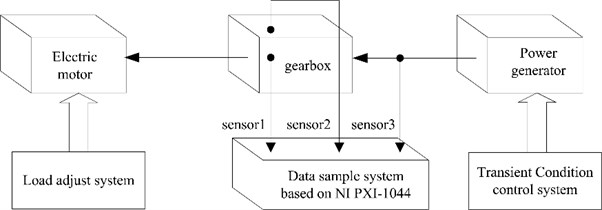Fig. 2. Real platform of experiment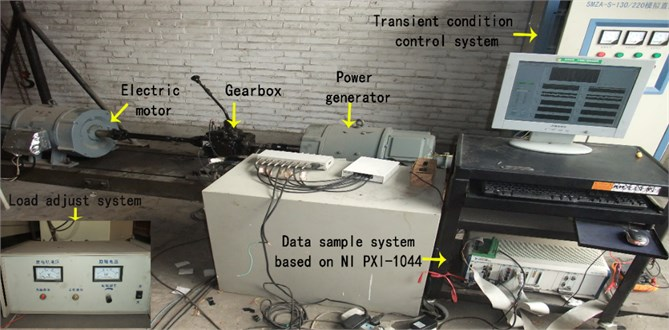#### 3.2. Diagnose bearing’s fault by TEEPAD

The signal of the acceleration and deceleration process is sampled as bearing under the normal, outside exfoliation, inside exfoliation and concurrent exfoliation conditions, and is resampled by equal angle method to stationary signal in angular domain, which is analyzed by Teager demodulation spectrum (TDS) and shown in Fig. 3.

It can be seen clearly from Fig. 3 that signal resampled at constant angle (SRCA) have no clear periodic impact signal, and feature order or its twice order are not found in the TDS of fault signal, which shows that feature of bearing’s early fault is so weak and submerged by other components, so it is difficult to be extracted by traditional time-domain method and TDS.

Fig. 3. SRCA and TDS of four conditions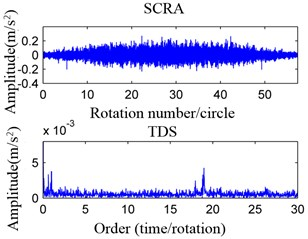a) Normal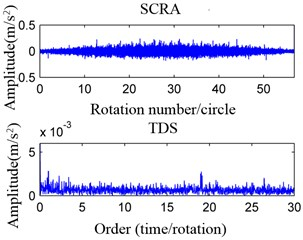b) Outside exfoliation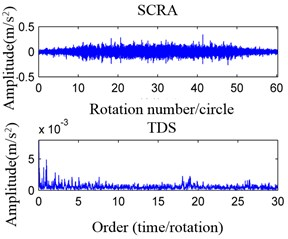c) Inside exfoliation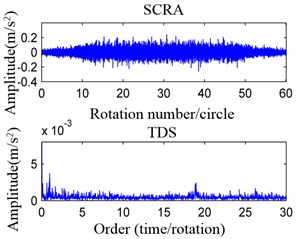d) Concurrent exfoliation

The signal of bearing under four conditions is analyzed by TEEPAD taking outside exfoliation feature number of circle (${R}_{out}$) and inside exfoliation feature number of circle (${R}_{in}$) as period, and the result is shown in Fig. 4.

Fig. 4. TEEPAD analysis of single and concurrent fault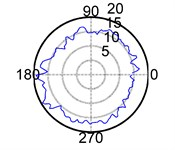a) Normal $Rout=$ 0.88496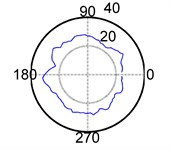b) Normal $Rin=$ 0.53191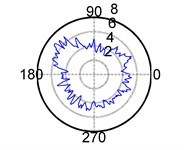c) Outside exfoliation $Rout=$ 0.88496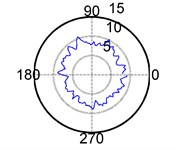d) Outside exfoliation $Rin=$ 0.53191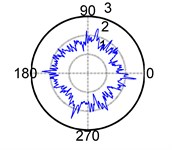e) Inside exfoliation $Rout=$ 0.75758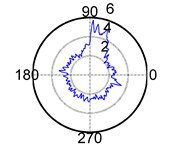f) Inside exfoliation $Rin=$ 0.4717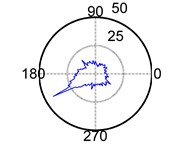g) Concurrent exfoliation $Rout=$ 0.75758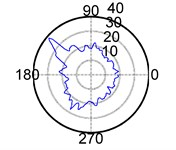h) Concurrent exfoliation $Rin=$ 0.4717

It can be seen clearly from Fig. 4(a) and Fig. 4(b) that normal signal is not enhanced on polar coordinate plane of ${R}_{out}$ and ${R}_{in}$, which shows that there are no fault on bearing; comparison of Fig. 4(c) and Fig. 4(d) shows that signal is enhanced only on polar coordinate plane of ${R}_{out}$, which shows that there is outside exfoliation on bearing; comparison of Fig. 4(e) and Fig. 4(f) shows that signal is enhanced only on the polar coordinate plane of ${R}_{in}$, which shows that there is inside exfoliation on bearing; comparison of Fig. 4(g) and Fig. 4(h) shows that signal is enhance both on the polar coordinate plane of ${R}_{out}$ and ${R}_{in}$, which shows that there are inside exfoliation and outside exfoliation on bearing at the same time. Taken together, periodic components can be enhanced to a higher amplitude scale, but the other components and noise will be restrained to a lower amplitude scale, so TEEPAD is able to enhance and detect weak fault impact feature created by early fault effectively.

#### 4. Conclusions

(1) Weak feature of bearing’s early fault can be exposed by the acceleration and deceleration process of transmission, and the unstable signal of variable rotation speed process can be analyzed effectively by TEEPAD.

(2) TEEPAD can restrain other components with different period and random noise, remove disturbance of concurrent fault’s modulate source, and extract fault feature of bearing’s single or concurrent fault.

1. Cao Ying, Duan Yubo, Liu Jicheng. Research and application of mode mixing in Hilbert-Huang transform. Journal of Vibration, Measurement and Diagnosis, Vol. 36, Issue 3, 2016, p. 518-523. [Search CrossRef]
2. Sun L., Zhang L., Du B., et al. Rolling bearing fault diagnosis based on improved wavelet threshold de-noising method and Teager energy operator. North China Electric Power, 2017. [Search CrossRef]
3. Bo S., Tian F., Jian T., et al. Fault diagnosis of rolling bearing based on Teager energy operator and EEMD. Journal of Beijing University of Technology, Vol. 43, Issue 6, 2017, p. 859-864. [Search CrossRef]
4. Elbouchikhi E., Choqueuse V., Amirat Y., et al. An efficient Hilbert-Huang transform-based bearing faults detection in induction machines. IEEE Transactions on Energy Conversion, Vol. 32, Issue 2, 2017, p. 401-413. [Publisher]
5. Soualhi A., Medjaher K., Zerhouni N., et al. Early detection of bearing faults by the Hilbert-Huang transform. International Conference on Control Engineering and Information Technology, 2017. [Publisher]
6. Wang Tian Jin, Feng Zhi Peng, Hao Ru Jiang. Fault diagnosis of rolling element bearing based on Teager energy operator. Journal of Vibration and Shock, Vol. 31, Issue 2, 2012, p. 85. [Search CrossRef]
7. Zhu Zhong Kui, Wu Xiao Yan, Li De Feng. Synchronous enhancement of periodic transient feature based on polar diagram for bearing diagnosis. Journal of Vibration Engineering, Vol. 21, Issue 6, 2008, p. 635-638. [Search CrossRef]
8. Ju Ping Hua, Qin Shu Ren, Zhao Ling. Energy operator demodulating approach based on LMD and its application in extracting characteristics of a fault signal. Journal of Vibration and Shock, Vol. 30, Issue 2, 2010, p. 1-4. [Search CrossRef]
9. Feng Z., Zuo M. J., Hao R., et al. Ensemble empirical mode decomposition-based Teager energy spectrum for bearing fault diagnosis. Journal of Vibration and Acoustics-Transactions, Vol. 135, Issue 3, 2013, p. 31013. [Publisher]
10. Li H., Fu L., Zhang Y. Bearing faults diagnosis based on teager energy operator demodulation technique. International Conference on Measuring Technology and Mechatronics Automation, 2009, p. 594-597. [Publisher]